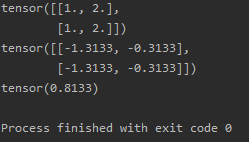I want to konw how can I get the loss=0.8133
could you please tell me the details such as which one multiply which one to get this result?
Thanks.

According to the formula in the doc:

``````sample 0:
- x + log ( sum(exp(x)) ) = 0.3133 + log( 0.2689 + 0.7310 ) = 0.3133 - 3.831e-5 = 0.3133
sample 1:
- x + log ( sum(exp(x)) ) = 1.3133 + log( 0.2689 + 0.7310 ) = 1.3133 - -3.831e-5 = 1.3133
average of the results:
(1.3133 + 0.3133)*0.5 = 0.8133
``````
1 Like

Thanks a lot.That’s very clear.